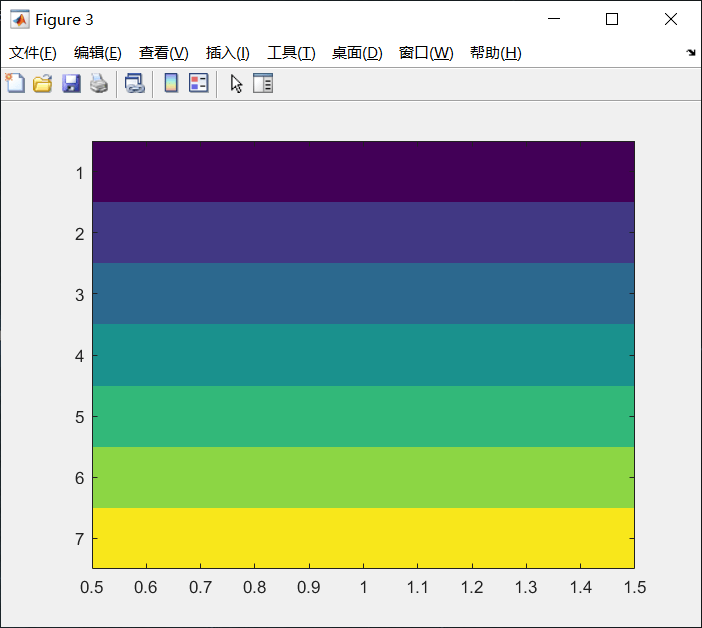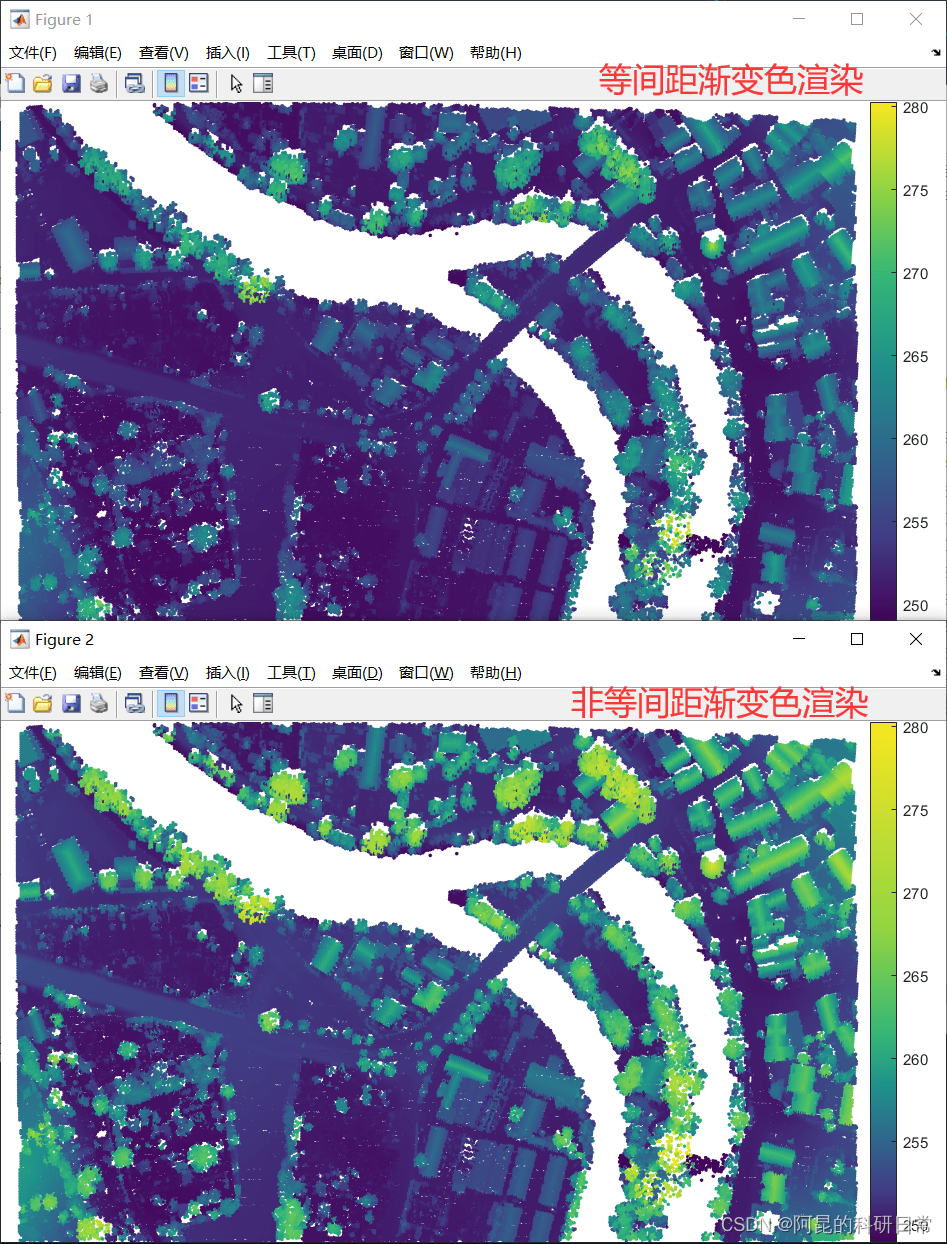# Matlab渐变颜色图(colormap)生成/编辑器—ColorMap为了解决上述问题，我用Matlab制作了这个ColorMap渐变色生成/编辑器

# 功能1：生成渐变色颜色图

``C = [  2  48  71``      18 104 131``      39 158 188``     144 201 230``     255 255 255]/255;````N = 256;``map = ColorMap(C,N);``# 功能2：致密化与稀疏化

``C = addcolorplus(300);``% 绘图``figure``image(permute(C,[1,3,2]))````% 致密化后渐变色总数``N1 = 256;``% ColorMap颜色致密化处理``map = ColorMap(C,N1);``% 绘图``figure``image(permute(map,[1,3,2]))````% 稀疏化后渐变色总数``N2 = 7;``% ColorMap颜色稀疏化处理``map = ColorMap(C,N2);``% 绘图``figure``image(permute(map,[1,3,2]))``# 功能3：显示区间调节

``C = [068 004 090``     065 062 133``     048 104 141``     031 146 139``     053 183 119``     145 213 066``     248 230 032]/255;````ratio = [0.1 0.1 0.1 0.1 0.2 0.4];``

``map2 = ColorMap(C,N,'mode2',ratio);``具体示例程序见demo3

# 获取方式：

Matlab渐变色生成器ColorMap